Do you like the article?
Share it with others —
Use new possibilities of MetaTrader 5

#### Similar articles# Graphical Control of External Parameters of Indicators

MetaTrader 4Examples | 8 July 2013, 10:045 7650### Introduction

How often do you have to set or change external parameters of indicators? Many of you must have once thought of moving them to the chart to be able to conveniently drag an icon or an object to the required position using the mouse, thus simplifying the indicator setup procedure. The MQL4 language allows for display of indicator settings on the screen.

### Graphical Control of Parameters

First of all, we need to find out what parameters there are in our indicator and which of them we are going to change. To do this, open the indicator properties and write down the names of parameters that will need to be changed in the course of work.

As an example, we use the ZigZag indicator included in the standard terminal delivery. Select the names of the variables in the properties.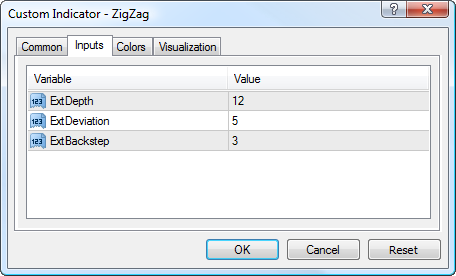Indicator Parameters

• ExtDepth=12
• ExtDeviation=5
• ExtBackstep=3

We further create variables and arrays that will be used to change indicator parameter values.

Names of the indicator parameters will be given in accordance with the parameters of the indicator which we are going to control.

```// Initial indicator values
int               ExtDepth=12;
int               ExtDeviation=5;
int               ExtBackstep=3;
//---- indicator buffers
double            ZigzagBuffer[];
double            HighMapBuffer[];
double            LowMapBuffer[];
// Array of indicator parameter variables
string            ArrExt[3,3]    ={"12","21","34", "5","8","21", "3","13","34"};
// Arrays of indices
string            Array_Index[]={"Dep -> ","Dev -> ","Back ->"};
int               Coordinats_IndexUp[]={65,160,260};
int               Coordinats_Index[]  ={35,130,230};
int               Coordinats_IndexDn[]={5, 100,200};
// Color for parameter blocks and indices
color             Color_[]={Blue,Green,Red};```

I believe, everything is clear at this stage.

`"Dep -> ","Dev -> ","Back ->"`

are ExtDepth, ExtDeviation and ExtBackstep, respectively. This is how they will be displayed in the chart.

For each of the parameters, we use three variable values

```string            ArrExtDepth[]    ={"12","15","20"};
string            ArrExtDeviation[]={"5",  "8","21"};
string            ArrExtBackstep[] ={"3", "13","34"};```

stored in a three-dimensional array for convenience:

`string            ArrExt[3,3]    ={"12","21","34", "5","8","21", "3","13","34"};`

3, 5, 12 are standard values set by default in the parameters of the standard ZigZag indicator.

Other values are given here as an example. They will be used as parameter variables for indicator control.

Considering that there will be a lot of plotting involved, we put a function for creating and moving objects into a separate block:

```void Obj_CreateMove_Text (int CreateMove, string Name, int Type, string Number,  color text_color,
int DistX, int DistY)
{
if (CreateMove==0)
ObjectCreate(Name,Type,0,0,0);
ObjectSetText(Name,Number,10,"Arial",text_color);
ObjectSet(Name,101,3);
ObjectSet(Name, 102, DistX);
ObjectSet(Name, 103, DistY);
}```

To ensure ease of control and in order to keep the code compact, all coordinates of the graphical objects are stored in arrays. This has already been demonstrated above (see the beginning of the article).

Let's now plot all the objects in the chart window.

The right hand side of our chart is less loaded with information so the variables will be arranged vertically along the right border of the window. Each parameter has its own index whose color will be the same as the color of corresponding parameter variables.

Thus, we need to create three parameter indices and three variable values for each of the parameters.

Create objects for the indicator parameters on the screen, display numerical values of variables for these parameters and create the corresponding indices next to each parameter block.

```// Create objects
for( ii=0;ii<3;ii++)
for( i=0;i<3;i++)
Obj_CreateMove_Text (0, Array_Index[ii]+i, 23, (ArrExt[ii,i]), Color_[ii], 5, Coordinats_IndexDn[ii]+30*i);
for( i=0;i<3;i++)
Obj_CreateMove_Text (0, "Index"+Array_Index[i], 23, (Array_Index[i]),   Color_[i], 25, Coordinats_Index[i]);```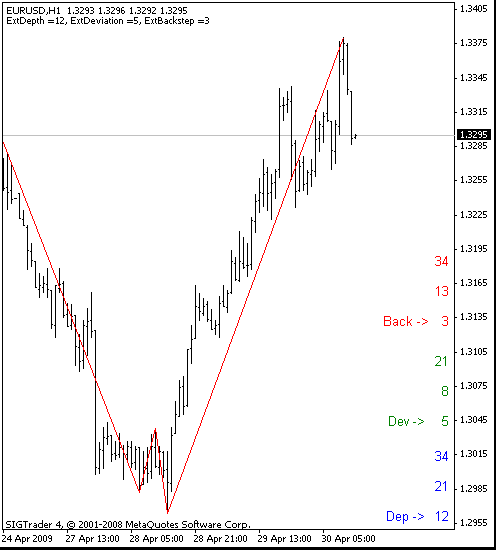Figure 1

Now we only need to create a block for handling user actions when the relevant index is moved.

We have anchor points of the objects on the chart according to which we read the index parameters one by one and determine the program logic.

```// Read index coordinates from the chart
for( i=0;i<3;i++)
{
Coordinats[i]=ObjectGet("Index"+Array_Index[i],OBJPROP_YDISTANCE);
if (Coordinats[i]==0)//If outside of the chart, set again
Obj_CreateMove_Text (1, "Index"+Array_Index[i], 23, Array_Index[i], Color_[i], 25, Coordinats_IndexDn[i]);
}    ```

Now that the obtained values have been saved, we need to analyze their positions on the chart and do the necessary. For the analysis, we use the actual positions of indices, taking as a reference point three base values of each of the indices relative to the values of the parameters used. If the index is moved above the highest value in its group, it will be deemed to have been moved up to its highest value. If the index is moved below the lowest parameter value, it will similarly be moved to the lowest parameter value. If the index is between the highest and lowest values of the parameter, it takes the middle value. For comparison purposes, we use a vertical scale, i.e.

`the OBJPROP_YDISTANCE parameter`

from

`ObjectGet()`
```// Analyze positions of indices
for( i=0;i<3;i++)
{
if (Coordinats[i]>Coordinats_IndexUp[i])
{Move[i] = Coordinats_IndexUp[i]; index[i]=2;}// Index has been moved up
if (Coordinats[i]>Coordinats_IndexDn[i]  &&
Coordinats[i]<Coordinats_IndexUp[i])
{Move[i] = Coordinats_Index[i];   index[i]=1;}// Index is in the middle
if (Coordinats[i]<Coordinats_IndexDn[i])
{Move[i] = Coordinats_IndexDn[i]; index[i]=0;}// Index has been moved down
if (Move[i]!=0)```

Then we align the objects that have been moved.

Save the new parameter values for plotting ZigZag.

Plot the indicator based on the values set using the graphical control.

```// Move and align the objects depending on their positions
Obj_CreateMove_Text (1, "Index"+Array_Index[i], 23, Array_Index[i], Color_[i], 25, Move[i]);
// Save ZigZag parameter values
switch (i)
{
case 0:  ExtDepth =     StrToInteger(ArrExt[0,index[i]]);  break;
case 1:  ExtDeviation = StrToInteger(ArrExt[1,index[i]]);  break;
case 2:  ExtBackstep =  StrToInteger(ArrExt[2,index[i]]);  break;
}/**/
}
// Pass parameter values to the custom indicator and plot ZigZag based on the obtained values
for(i=300;i>0;i--)
{
ZigzagBuffer[i]=iCustom(NULL,0,"ZigZag",ExtDepth,ExtDeviation,ExtBackstep,0,i);
HighMapBuffer[i]=iCustom(NULL,0,"ZigZag",ExtDepth,ExtDeviation,ExtBackstep,1,i);
LowMapBuffer[i]=iCustom(NULL,0,"ZigZag",ExtDepth,ExtDeviation,ExtBackstep,2,i);
}```

Double-click on any graphical object to activate it. When activated, the object can be controlled using the mouse. This needs to be done every time you decide to change the parameter value.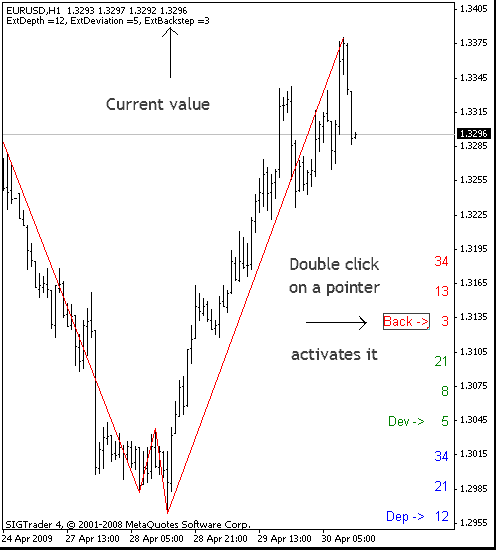Figure 2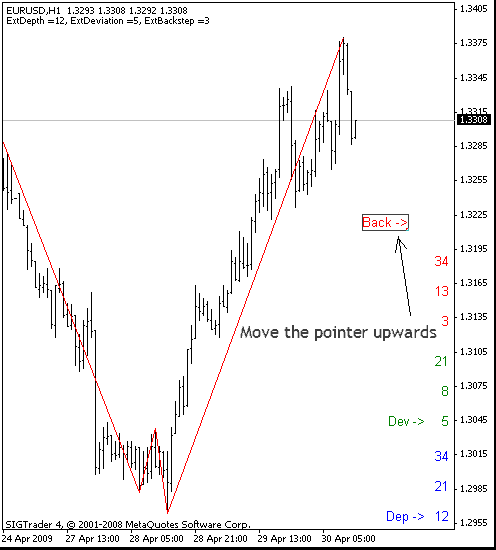Figure 3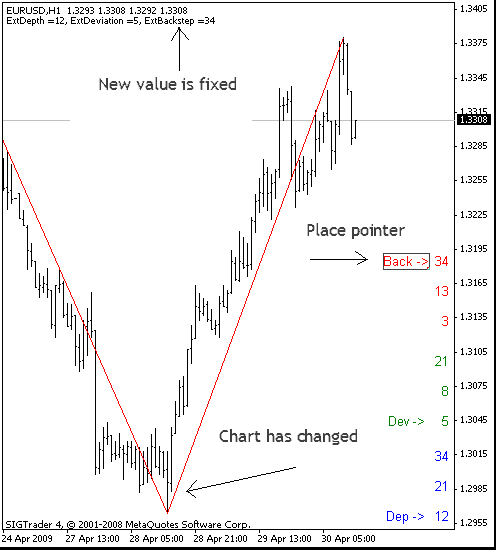Figure 4

The final version of the code will be as follows.

```//+------------------------------------------------------------------+
//|                                         management_indicator.mq4 |
//|                                             http://www.fibook.ru |
//+------------------------------------------------------------------+

#property indicator_chart_window
#property indicator_buffers 1
#property indicator_color1 Red
// Initial indicator values
int               ExtDepth=12;
int               ExtDeviation=5;
int               ExtBackstep=3;
//---- indicator buffers
double            ZigzagBuffer[];
double            HighMapBuffer[];
double            LowMapBuffer[];
// Array of indicator parameter variables
string            ArrExt[3,3]    ={"12","21","34", "5","8","21", "3","13","34"};
// Arrays of indices
string            Array_Index[]={"Dep -> ","Dev -> ","Back ->"};
int               Coordinats_IndexUp[]={65,160,260};
int               Coordinats_Index[]  ={35,130,230};
int               Coordinats_IndexDn[]={5, 100,200};
// Color for parameter blocks and indices
color             Color_[]={Blue,Green,Red};
// Variables for calculations
int               i,ii;
int               index;
//+------------------------------------------------------------------+
//| Custom indicator initialization function                         |
//+------------------------------------------------------------------+
int init()
{
//---- indicators
IndicatorBuffers(3);
//---- drawing settings
SetIndexStyle(0,DRAW_SECTION);
//---- indicator buffers mapping
SetIndexBuffer(0,ZigzagBuffer);
SetIndexBuffer(1,HighMapBuffer);
SetIndexBuffer(2,LowMapBuffer);
SetIndexEmptyValue(0,0.0);
//----
return(0);
}
//+------------------------------------------------------------------+
//| Custom indicator deinitialization function                       |
//+------------------------------------------------------------------+
int deinit()
{
//----

//----
return(0);
}
//+------------------------------------------------------------------+
//| Custom indicator iteration function                              |
//+------------------------------------------------------------------+
int start()
{
int  Coordinats;
int  Move;
// Check if index objects are on the chart
if (ObjectGet("IndexDep -> ",OBJPROP_YDISTANCE)<1||
ObjectGet("IndexDev -> ",OBJPROP_YDISTANCE)<1||
ObjectGet("IndexBack ->",OBJPROP_YDISTANCE)<1)
// If they are not on the chart, create them
{
for( ii=0;ii<3;ii++)
for( i=0;i<3;i++)
Obj_CreateMove_Text (0, Array_Index[ii]+i, 23, ArrExt[ii,i], Color_[ii], 5, Coordinats_IndexDn[ii]+30*i);
for( i=0;i<3;i++)
Obj_CreateMove_Text (0, "Index"+Array_Index[i], 23, Array_Index[i], Color_[i], 25, Coordinats_IndexDn[i]);
}
// Read index coordinates from the chart
for( i=0;i<3;i++)
{
Coordinats[i]=ObjectGet("Index"+Array_Index[i],OBJPROP_YDISTANCE);
if (Coordinats[i]==0)//If outside of the chart, set again
Obj_CreateMove_Text (1, "Index"+Array_Index[i], 23, Array_Index[i], Color_[i], 25, Coordinats_IndexDn[i]);
}
// Analyze positions of indices
for( i=0;i<3;i++)
{
if (Coordinats[i]>Coordinats_IndexUp[i])
{Move[i] = Coordinats_IndexUp[i]; index[i]=2;}//Index has been moved up
if (Coordinats[i]>Coordinats_IndexDn[i]  &&
Coordinats[i]<Coordinats_IndexUp[i])
{Move[i] = Coordinats_Index[i];   index[i]=1;}// Index is in the middle
if (Coordinats[i]<Coordinats_IndexDn[i])
{Move[i] = Coordinats_IndexDn[i]; index[i]=0;}// Index has been moved down
if (Move[i]!=0)
// Move and align the objects depending on their positions
Obj_CreateMove_Text (1, "Index"+Array_Index[i], 23, Array_Index[i], Color_[i], 25, Move[i]);
// Save ZigZag parameter values
switch (i)
{
case 0:  ExtDepth =     StrToInteger(ArrExt[0,index[i]]);  break;
case 1:  ExtDeviation = StrToInteger(ArrExt[1,index[i]]);  break;
case 2:  ExtBackstep =  StrToInteger(ArrExt[2,index[i]]);  break;
}/**/
}
// Pass parameter values to the custom indicator and plot ZigZag based on the obtained values
for(i=300;i>0;i--)
{
ZigzagBuffer[i]=iCustom(NULL,0,"ZigZag",ExtDepth,ExtDeviation,ExtBackstep,0,i);
HighMapBuffer[i]=iCustom(NULL,0,"ZigZag",ExtDepth,ExtDeviation,ExtBackstep,1,i);
LowMapBuffer[i]=iCustom(NULL,0,"ZigZag",ExtDepth,ExtDeviation,ExtBackstep,2,i);
}
Comment ("ExtDepth =",ExtDepth,", ExtDeviation =",ExtDeviation,", ExtBackstep =",ExtBackstep);
//----
return(0);
}
// Function for creating and moving graphical objects
void Obj_CreateMove_Text (int CreateMove, string Name, int Type, string Number,  color text_color, int DistX, int DistY)
{
if (CreateMove==0)
ObjectCreate(Name,Type,0,0,0);
ObjectSetText(Name,Number,10,"Arial",text_color);
ObjectSet(Name,101,3);
ObjectSet(Name, 102, DistX);
ObjectSet(Name, 103, DistY);
}

//+------------------------------------------------------------------+```

### Conclusion

The approach described above can be implemented to control any indicator externally by displaying the parameters that need to be set.

Translated from Russian by MetaQuotes Ltd.
Original article: https://www.mql5.com/ru/articles/1370

#### Other articles by this author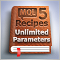MQL5 Cookbook: Developing a Multi-Currency Expert Advisor with Unlimited Number of Parameters
In this article, we will create a pattern that uses a single set of parameters for optimization of a trading system, while allowing for unlimited number of parameters. The list of symbols will be created in a standard text file (*.txt). Input parameters for each symbol will also be stored in files. This way we will be able to circumvent the restriction of the terminal on the number of input parameters of an Expert Advisor.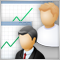Social Trading with the MetaTrader 4 and MetaTrader 5 Trading Platforms
What is social trading? It is a mutually beneficial cooperation of traders and investors whereby successful traders allow monitoring of their trading and potential investors take the opportunity to monitor their performance and copy trades of those who look more promising.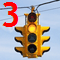Alert and Comment for External Indicators. Multi-Currency Analysis Through External Scanning
Alert for multi-currency and multiple time frame analysis of external indicators. The article deals with a method of getting event information in respect of events in external indicators, without having to attach indicators to a chart or open charts themselves. We will call it external scanning.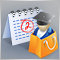MQL5 Market Results for Q2 2013
Successfully operating for 1.5 years, MQL5 Market has become the largest traders' store of trading strategies and technical indicators. It offers around 800 trading applications provided by 350 developers from around the world. Over 100.000 trading programs have already been purchased and downloaded by traders to their MetaTrader 5 terminals.